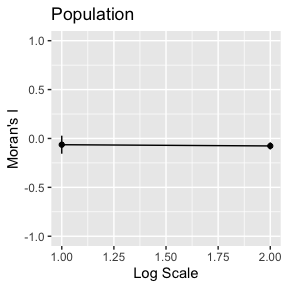# Moran’s I rescaling

#### 2019-11-21

Many Geographical Analysis utilizes spatial autocorrelation, that allows us to study the geographical evolution from different points of view. One measurement for spatial autocorrelation is Moran’s I, that is based on Pearson’s correlation coefficient in general statistics (Chen 2013)

## Performing the Analysis

This package offers two ways to rectify Moran’s I. The first method is a Procrustes method, and the second one is a rectifying through Pearson correlation. We provided a straight forward way to perform both techniques.

### Rectification method 1. Three-point anisometric scaling (3-PAS)

invited every passer-by to spend the night. If the guess were too short, Procrustes would stretch him to fit, or If the guest proved too tall, Procrustes would amputate the excess length. Keeping Procrustes’s myth in mind, what this technique does is to stretch or cut as necessary to make the Moran’s I null distribution to fit a Normal Curve, making the max and min or 99.99% - 0.1% quantile, values to match.

The package offers, as stated before, a direct way to apply the Procrustes technique to a data set. The function rescaleI requires an input file path, the number of samples to create the null distribution, and a parameter that establishes up to which value the Moran’s I value will be stretch.

The Loading data section explains the required format of the input file. The parameter scalingUpTo accepts two string values, “MaxMin” and “Quantile.” The first value is self-explanatory, while the second one refers to the quantiles 0.1% and 99.99%. The default value is “Quantile”, meaning that, if something different to “MaxMin” or “Quantile” is sent, the iCorrection function will apply “Quantile”.

library(Irescale)
fileInput<-system.file("testdata", "chen.csv", package="Irescale")
scaledI<-rescaleI(data,samples=1000, scalingUpTo="MaxMin")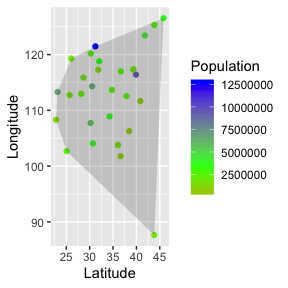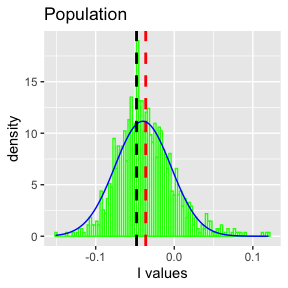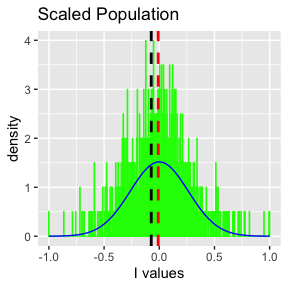fn = file.path(tempdir(),"output.csv",fsep = .Platform$file.sep) saveFile(fn,scaledI) if (file.exists(fn)) #Delete file if it exists file.remove(fn) #>  TRUE ### Rectified Correlation Three-point anisometric scaling (3-PAS) method of rectification eliminated negative bias and more closely approached the theoretical distribution of regular (Pearson, Spearman) correlations, hence intuitive expectations for such; however, distribution shape depended upon choice of critical percentiles and did not conform to intuitive expectations for Gaussian shape. These results inspired development of a second approach which mapped$ $values, based on their quantiles, to the theoretical values of regular correlations expected for each quantile. This latter method yielded perfectly repeatable null distributions that were Gauss-like but with definitive limits at$ 1$fileInput <- system.file("testdata", "chen.csv", package="Irescale") data <- loadFile(fileInput) rectifiedI<-rectifyIrho(data,1000)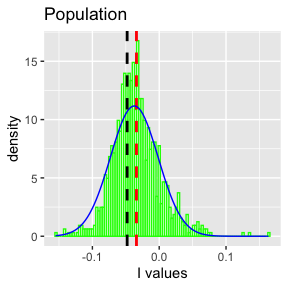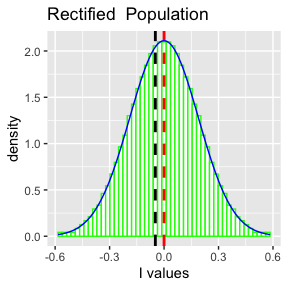fn = file.path(tempdir(),"output.csv",fsep = .Platform$file.sep)
saveFile(fn,rectifiedI)
if (file.exists(fn))
#Delete file if it exists
file.remove(fn)
#>  TRUE

## Analysis Step by Step

The method rescaleI is a wrap for a pipeline that can be executed step by step.

The input file1 should have the following format.

• The first column represents an unique id for the record.
• The second and third column represent the latitute and longitud of where the sample was taken
• The fourth and beyond represents the different measured variables
fileInput<-system.file("testdata", "chen.csv", package="Irescale")
#>           City Latitude Longitude Population
#> 1      Beijing 39.90420  116.4074    9496688
#> 2      Tianjin 39.34336  117.3616    5313702
#> 3 Shijiazhuang 39.34336  117.3616    1930579
#> 4      Taiyuan 37.87059  112.5489    2538336
#> 5      Hohehot 40.82192  111.6581     990954
#> 6     Shenyang 41.80570  123.4315    4344933

To load data to performe the analysis is quite simple. The function loadFile provides the interface to make it. loadFile returns a list with two variables, data and varOfInterest, the first one represents a vector with latitude and longitude; varOfInterest is a matrix with all the measurements from the field.

library(Irescale)
fileInput<-system.file("testdata", "chen.csv", package="Irescale")
head(input$data) #> Latitude Longitude #> Beijing 39.90420 116.4074 #> Tianjin 39.34336 117.3616 #> Shijiazhuang 39.34336 117.3616 #> Taiyuan 37.87059 112.5489 #> Hohehot 40.82192 111.6581 #> Shenyang 41.80570 123.4315 head(input$varOfInterest)
#>              Population
#> Beijing         9496688
#> Tianjin         5313702
#> Shijiazhuang    1930579
#> Taiyuan         2538336
#> Hohehot          990954
#> Shenyang        4344933

If the data has a chessboard shape,the file is organized in rows and columns, where the rows represent latitute and columns longitude, the measurements are in the cell. The function loadChessBoard can be used to load into the analysis.

library(Irescale)
fileInput<-"../inst/testdata/chessboard.csv"
head(input$data) #> [,1] [,2] #> [1,] 1 1 #> [2,] 1 2 #> [3,] 1 3 #> [4,] 1 4 #> [5,] 1 5 #> [6,] 1 6 head(input$varOfInterest)
#>  1 1 1 1 1 1

### Calculate Distance

Once the data is loaded, The distance matrix, the distance between all the points might be calcualted. The distance can be calculated using calculateEuclideanDistance’ if the points are taken in a geospatial location.

library(Irescale)
fileInput<-system.file("testdata", "chen.csv", package="Irescale")
distM<-calculateEuclideanDistance(input$data) distM[1:5,1:5] #> [,1] [,2] [,3] [,4] [,5] #> [1,] 0.000000 1.106865 1.106865 4.361616 4.837197 #> [2,] 1.106865 0.000000 0.000000 5.033068 5.892130 #> [3,] 1.106865 0.000000 0.000000 5.033068 5.892130 #> [4,] 4.361616 5.033068 5.033068 0.000000 3.082845 #> [5,] 4.837197 5.892130 5.892130 3.082845 0.000000 If the data is taken from a chessboard a like field, the Manhattan distance can be used. library(Irescale) fileInput<-"../inst/testdata/chessboard.csv" input<-loadChessBoard(fileInput) distM<-calculateManhattanDistance(input$data)
distM[1:5,1:5]
#>      [,1] [,2] [,3] [,4] [,5]
#> [1,]    0    1    2    3    4
#> [2,]    1    0    1    2    3
#> [3,]    2    1    0    1    2
#> [4,]    3    2    1    0    1
#> [5,]    4    3    2    1    0

### Calculate Weighted Distance Matrix

The weighted distance matrix can be calculated it using the function calculateWeightedDistMatrix, however it is not required to do it, because ‘calculateMoranI’ does it.

library(Irescale)
fileInput<-system.file("testdata", "chen.csv", package="Irescale")
I<-calculateMoranI(distM = distM,varOfInterest = input$varOfInterest) I #>  -0.04800907 ### Resampling Method for I The scaling process is made using Monte Carlo resampling method. The idea is to shuffle the values and recalculate I for at least 1000 times. In the code below, after resampling the value of I, a set of statistics are calculated for that generated vector. library(Irescale) fileInput<-system.file("testdata", "chen.csv", package="Irescale") input<-loadFile(fileInput) distM<-calculateEuclideanDistance(input$data)
I<-calculateMoranI(distM = distM,varOfInterest = input$varOfInterest) vI<-resamplingI(distM, input$varOfInterest) # This is the permutation
statsVI<-summaryVector(vI)
statsVI
#> $mean #>  -0.03531522 #> #>$sd
#>  0.03654939
#>
#> $max #>  0.2290524 #> #>$min
#>  -0.1350819
#>
#> $Q1 #> 0.1% #> -0.1321211 #> #>$Q99
#>    99.99%
#> 0.2208389
#>
#> $median #>  -0.03868094 #> #>$skew
#>  1.009807
#>
#> $kurt #>  3.973344 #### Plotting Distribution (Optional) To see how the value of I is distribuited, the method plotHistogramOverlayNormal provides the functionality to get a histogram of the vector generated by resampling with a theorical normal distribution overlay. library(Irescale) fileInput<-system.file("testdata", "chen.csv", package="Irescale") input<-loadFile(fileInput) distM<-calculateEuclideanDistance(input$data)
I<-calculateMoranI(distM = distM,varOfInterest = input$varOfInterest) vI<-resamplingI(distM, input$varOfInterest) # This is the permutation
statsVI<-summaryVector(vI)
plotHistogramOverlayNormal(vI,statsVI, main=colnames(input$varOfInterest))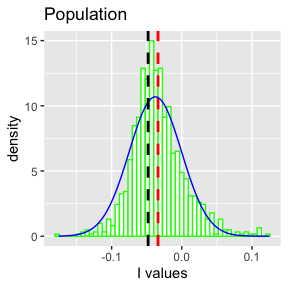### Rectifying I Once we have calculated the null distribution via resampling, you need to scale by centering and streching. The method iCorrection, return an object with the resampling vector rescaled, and all the summary for this vector, the new value of I is returned in a variable named newI #### Rectification method 1. Three-point anisometric scaling (3-PAS) As a reminder there are two ways to rectify I, in this section we present the procrustes methodology. library(Irescale) fileInput<-system.file("testdata", "chen.csv", package="Irescale") input<-loadFile(fileInput) distM<-calculateEuclideanDistance(input$data)
I<-calculateMoranI(distM = distM,varOfInterest = input$varOfInterest) vI<-resamplingI(distM, input$varOfInterest) # This is the permutation
statsVI<-summaryVector(vI)
corrections<-iCorrection(I,vI)
corrections$newI #> 0.1% #> -0.1050817 #### Rectification method 2. Pearson Correlation fileInput <- system.file("testdata", "chen.csv", package="Irescale") data <- loadFile(fileInput) distM<-calculateEuclideanDistance(data$data)
vI<-resamplingI(distM,data$varOfInterest,n = 100000) rectifiedI<- ItoPearsonCorrelation(vI, length(data)) rectifiedI$newI
#>  -0.3301686

## Calculate P-value

In order to provide a significance to this new value, you can calculate the pvalue using the method calculatePvalue. This method requires the scaled vector, you get this vector,scaledData, the scaled I, newI and the mean of the scaledData.

library(Irescale)
fileInput<-system.file("testdata", "chen.csv", package="Irescale")
distM<-calculateEuclideanDistance(input$data) I<-calculateMoranI(distM = distM,varOfInterest = input$varOfInterest)
vI<-resamplingI(distM, input$varOfInterest) # This is the permutation statsVI<-summaryVector(vI) corrections<-iCorrection(I,vI) pvalueIscaled<-calculatePvalue(corrections$scaledData,corrections$newI,corrections$summaryScaledD\$mean)
pvalueIscaled
#>  0.3606394

## Stability Analysis

In order to determine how many iterations it is necessary to run the resampling method, it is possible to run a stability analysis. This function draw a chart in log scale (10^x) of the number of interations needed to achieve the stability in the Monte Carlo simulation. ### Rectifying to “Max-Min”

library(Irescale)
fileInput<-system.file("testdata", "chen.csv", package="Irescale")
resultsChen<-buildStabilityTable(data=input, times=10, samples=10^2, plots=TRUE)
#>  29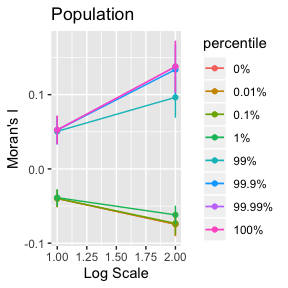### Rectifying with correlation

fileInput <- system.file("testdata", "chen.csv", package="Irescale")
resultsChen<-buildStabilityTableForCorrelation(data=data,times=10,samples=10^2,plots=TRUE)`